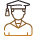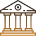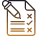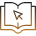# Mathematical Models In Epidemiology: The Covid-19 Pandemic

###### ByRamraj Saini###### Synopsis

How does maths play an important role in understanding the spread of Covid-19 diseases, predict the number of cases and evaluate the effectiveness of interventions such as social distancing and vaccination? How did it help in identifying populations at high risk of infection using various mathematical models such as SIR and SEIR models. To gain more in-depth knowledge, read the article.###### Synopsis

How does maths play an important role in understanding the spread of Covid-19 diseases, predict the number of cases and evaluate the effectiveness of interventions such as social distancing and vaccination? How did it help in identifying populations at high risk of infection using various mathematical models such as SIR and SEIR models. To gain more in-depth knowledge, read the article.

The Covid-19 pandemic has affected the world in unprecedented ways. As the virus continues to spread, it has become increasingly important to understand its dynamics and predict its future trajectory. This is where mathematical models come in. Mathematical models are tools that help us understand the spread of diseases, predict the number of cases, evaluate the effectiveness of interventions such as social distancing and vaccination, and identify populations at high risk of infection.

The study of mathematical models in epidemiology is an important topic in the science curriculum for Class 12 students. In the NCERT textbook for Class 12 biology, the chapter on "Human Health and Disease" covers the basic concepts of epidemiology, including the spread of infectious diseases and the principles of disease control. Mathematical models are briefly mentioned in this chapter as tools for understanding the spread of diseases and predicting their impact.

Studying mathematical models in epidemiology can help students develop critical thinking and problem-solving skills, as well as an appreciation for the power of mathematics in addressing real-world problems. These models are also relevant to a wide range of fields, including medicine, public health, and policy-making. By understanding these models, students can gain a deeper understanding of the complex interplay between biological, social, and environmental factors that influence the spread of infectious diseases.

Here in this article, we will discuss mathematical models in epidemiology particularly for Covid-19 including the concepts of transmission dynamics, forecasting the number of cases, and effectiveness of interventions.

## Transmission Of Covid-19

Mathematical models have been used extensively to study the transmission dynamics of Covid-19. These models aim to understand how the virus spreads from one person to another and how it can be controlled. One of the most commonly used mathematical models for Covid-19 transmission dynamics is the SIR model.

The SIR model divides the population into three compartments: Susceptible (S), Infected (I), and Recovered (R). The model assumes that individuals move from the susceptible compartment to the infected compartment, and from the infected compartment to the recovered compartment.

The basic idea behind the SIR model is that the rate of new infections is proportional to the number of susceptible individuals and the number of infected individuals. The formula for the SIR model is:

dS/dt = -βSI/N

dI/dt = βSI/N - γI

dR/dt = γI

Where S is the number of susceptible individuals, I is the number of infected individuals, R is the number of recovered individuals, β is the transmission rate, γ is the recovery rate, and N is the total population.

The transmission rate β represents the average number of people that an infected individual infects per day. The recovery rate γ represents the rate at which infected individuals recover and become immune to the virus. The formula for the basic reproduction number (R0) in the SIR model is:

R0 = β/γ

If R0 is greater than 1, then the virus will spread through the population. If R0 is less than 1, then the virus will eventually die out.

For example, suppose the transmission rate β is 0.2, the recovery rate γ is 0.05, and the total population N is 100,000. Then, the basic reproduction number R0 is:

R0 = β/γ = 0.2/0.05 = 4

This means that on average, each infected person will infect four others. If no interventions are put in place, the virus will continue to spread until a large portion of the population becomes immune.

Another commonly used mathematical model for Covid-19 transmission dynamics is the SEIR model. The SEIR model is similar to the SIR model, but it includes an additional compartment for individuals who are exposed to the virus but not yet infectious. The formula for the SEIR model is:

dS/dt = -βSI/N

dE/dt = βSI/N-αE

dI/dt = αE - γI

dR/dt = γI

Where S is the number of susceptible individuals, E is the number of exposed individuals, I is the number of infected individuals, R is the number of recovered individuals, β is the transmission rate, α is the incubation rate, and γ is the recovery rate.

The incubation rate α represents the rate at which individuals move from the exposed compartment to the infected compartment. The formula for the basic reproduction number (R0) in the SEIR model is:

R0 = β/(α + γ)

For example, suppose the transmission rate β is 0.2, the incubation rate α is 0.05, the recovery rate γ is 0.1, and the total population N is 100,000. Then, the basic reproduction number R0 is:

R0 = β/(α + γ) = 0.2/(0.05 + 0.1) = 1.33

This means that on average, each infected person will infect 1.33 others. If no interventions are put in place, the virus will persistently propagate until a significant proportion of the population has acquired immunity.

## Forecasting Covid-19 Cases

Mathematical models have also been used to forecast the number of cases for the Covid-19 pandemic. These models aim to predict the future trajectory of the pandemic. One of the most commonly used mathematical models for forecasting the number of cases of Covid-19 is the exponential growth model.

The exponential growth model assumes that cases grow exponentially over time. The formula for the exponential growth model is:

y = aebt

Where y is the number of cases, a is the initial number of cases, b is the growth rate, and t is the time.

For example, suppose the initial number of cases is 100, and the growth rate is 0.1. Then, the number of cases after 10 days is:

y = 100e0.1x10

y = 270

This means that the number of cases is expected to more than double in 10 days.

## Effectiveness Of Interventions

Mathematical models can also be used to evaluate the effectiveness of different interventions. For example, models can be used to estimate the impact of social distancing measures on the transmission of the virus. Such models can also be used to evaluate the impact of vaccination campaigns on the number of cases and deaths.

For example, the SEIR model discussed in the previous section can be used to forecast the number of cases by adjusting the transmission rate, incubation rate, and recovery rate based on the interventions put in place.

Now you are aware of the crucial role of mathematics in understanding the transmission of Covid-19, estimating case numbers, evaluating the efficacy of interventions like social distancing and vaccination, and identifying high-risk populations with mathematical models such as SIR and SEIR models.

## Careers360 helping shape your Career for a better tomorrow#### 250M+

Students#### 30,000+

Colleges#### 500+

ExamsE-Books